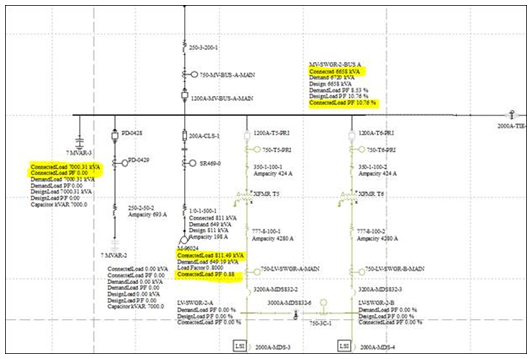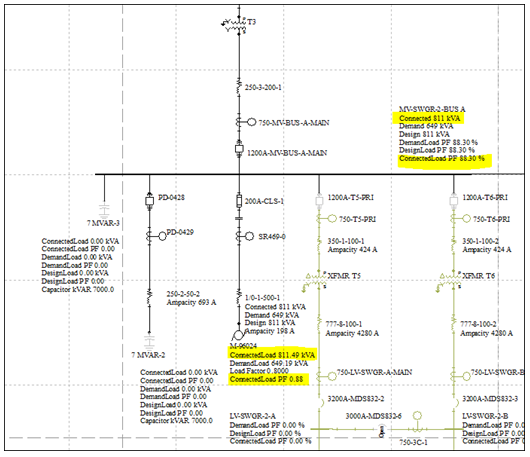• Sales
• Library Model Request
• Technical Support

# Why is the PF very low in running the Demand Load Study when a Capacitor is added to the system?

Question:
Why is the power factor (PF) very low in running the Demand Load Study when a Capacitor is added to the system?

Answer: The capacitor may have a large KVAR and providing most of the kVA which does not have real power (kW). See example below:Motor PF = 0.883
Capacitor size = 7000 kVAR, PF = 0

Using the equation P = S*PF:

Pmotor = Smotor*PFmotor = 811 * 0.883 = 716.11 kW
Pcapacitor = 0
Ptotal = Pmotor + Pcapacitor = 716.11 kW

Using the equation Q = S*sin(acos(PF))

Qmotor = Smotor*sin(acos(PFmotor)) = 811*sin(acos(0.883)) = 380.66 kVAR
Qcapacitor = Scapacitor*sin(acos(PFcapacitor)) = 7000*sin(acos(0)) = 7000 kVAR
Qtotal = Qmotor - Qcapacitor = -6619.34 kVAR

Stotal = sqrt (Ptotal^2 + Qtotal^2) = 6658 kVA

Calculating PF:
PF = Ptotal / Stotal = 716.11 / 6658 = 0.1076 --> 10.76%

Without the capacitor, Stotal = Smotor, thus PF = PF motor = 0.883 --> 88.3%